# 06-240/Classnotes For Thursday, September 28

## Contents

### Scan of Lecture notes

• Image file: week 3 lecture

### Linear Combination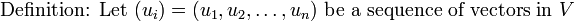$\mbox{Definition: Let }(u_i) = (u_1,u_2,\ldots,u_n)\mbox{ be a sequence of vectors in }V$.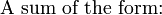$\mbox{A sum of the form:}{}_{}^{}$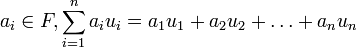$a_i\in F,\sum_{i=1}^n a_i u_i = a_1u_1 + a_2u_2+\ldots+a_nu_n$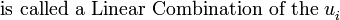$\mbox{is called a Linear Combination of the }u_i^{ }$.

### Span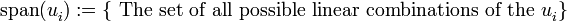$\mbox{span}(u_i^{ }):= \lbrace\mbox{ The set of all possible linear combinations of the } u_i^{ }\rbrace$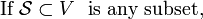$\mbox{If }\mathcal{S} \subset V\ \mbox{ is any subset, }$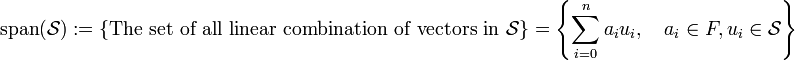$\mbox{span}(\mathcal{S}):= \lbrace\mbox{The set of all linear combination of vectors in }\mathcal{S}\rbrace=\left\lbrace\sum_{i=0}^n a_i u_i,\quad a_i \in F, u_i \in \mathcal{S}\right\rbrace$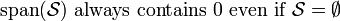$\mbox{span}(\mathcal{S})\mbox{ always contains }0\mbox{ even if }\mathcal{S}=\emptyset$

Theorem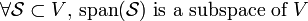$\forall\mathcal{S} \subset V\mbox{, span}(\mathcal{S})\mbox{ is a subspace of }V$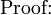$\mbox{Proof:}{}_{}^{}$

1.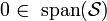$0 \in\mbox{ span}(\mathcal{S})$.
2.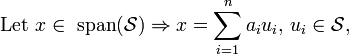$\mbox{Let }x \in \mbox{ span}(\mathcal{S})\Rightarrow x =\sum_{i=1}^n a_iu_i\mbox{, }u_i\in \mathcal{S}\mbox{, }$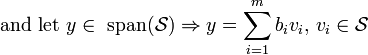$\mbox{and let }y \in \mbox{ span}(\mathcal{S})\Rightarrow y =\sum_{i=1}^m b_iv_i\mbox{, }v_i\in \mathcal{S}$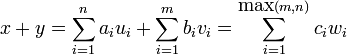$x+y = \sum_{i=1}^n a_iu_i+ \sum_{i=1}^m b_iv_i = \sum_{i=1}^{\mbox{max}(m,n)} c_iw_i$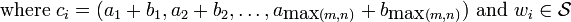$\qquad\mbox{ where }c_i=(a_1+b_1,a_2+b_2,\ldots,a_{\mbox{max}(m,n)}+b_{\mbox{max}(m,n)})\mbox{ and }w_i\in\mathcal{S}$

3.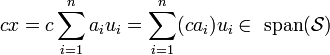$cx= c\sum_{i=1}^n a_iu_i=\sum_{i=1}^n(ca_i)u_i\in\mbox{ span}(\mathcal{S})$

Example 1.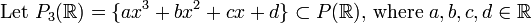$\mbox{Let } P_3(\mathbb{R})=\lbrace ax^3+bx^2+cx+d\rbrace\subset P(\mathbb{R})\mbox{, where }a, b, c, d \in \mathbb{R}$.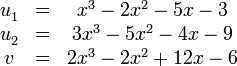$\begin{matrix}u_1^{}&=&x^3-2x^2-5x-3\\ u_2^{}&=&3x^3-5x^2-4x-9\\ v_{}^{}&=&2x^3-2x^2+12x-6\end{matrix}$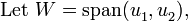$\mbox{Let }W=\mbox{span}(u_1^{},u_2^{})\mbox{,}$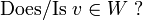$\mbox{Does/Is } v \in W\mbox{ ?}$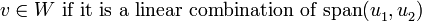$v\in W\mbox{ if it is a linear combination of span}(u_1^{},u_2^{})$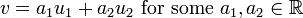$v=a_1u_1 + a_2u_2 \mbox{ for some }a_1, a_2 \in \mathbb{R}$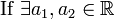$\mbox{If }\exists a_1,a_2\in \mathbb{R}$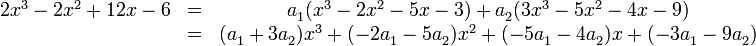$\begin{matrix}2x^3-2x^2+12x-6&=& a_1^{}(x^3-2x^2-5x-3) + a_2^{}(3x^3-5x^2-4x-9)\\ \ &=&(a_1^{}+3a_2^{})x^3 + (-2a_1^{}-5a_2^{})x^2 + (-5a_1^{}-4a_2^{})x + (-3a_1^{}-9a_2^{})\end{matrix}$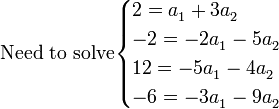$\mbox{Need to solve}\begin{cases} 2=a_1^{}+3a_2^{}\\ -2=-2a_1^{}-5a_2^{}\\ 12=-5a_1^{}-4a_2^{}\\ -6=-3a_1^{}-9a_2^{}\end{cases}$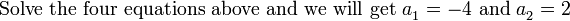$\mbox{Solve the four equations above and we will get }a_1^{}=-4\mbox{ and }a_2^{}=2$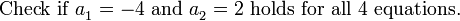$\mbox{Check if }a_1^{}=-4\mbox{ and }a_2^{}=2\mbox{ holds for all 4 equations.}$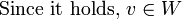$\mbox{Since it holds, } v\in W$